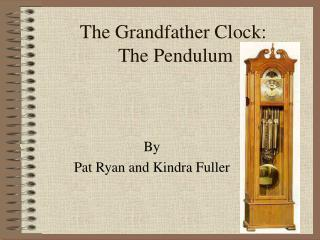DownloadDownload PresentationThe Grandfather Clock: The Pendulum

# The Grandfather Clock: The Pendulum

Download Presentation## The Grandfather Clock: The Pendulum

- - - - - - - - - - - - - - - - - - - - - - - - - - - E N D - - - - - - - - - - - - - - - - - - - - - - - - - - -
##### Presentation Transcript

1. The Grandfather Clock: The Pendulum By Pat Ryan and Kindra Fuller

2. Anchor

3. Amplitude • The angular displacement from its vertical or equilibrium position. It is measured in terms of the angle that it makes with the vertical.

4. Frequency • The number of cycles of a periodic process that occur per unit time (second). The unit of measure is the hertz (Hz).

5. Cycle • Cycle-one full motion from one amplitude position to the other and back again.

6. Period • Period-the time for one complete cycle of a periodic process and units are in seconds, minutes, etc.

7. Frequency Equation The equation to calculate the frequency of a pendulum is: f = (1/2pi) * sqrt(g/L) or f = (1/2π) * √(g/L)

8. Length of Pendulum If you wanted to find the length for a given frequency, you can write that equation as: L = g/(4*pi2*f2) or L = g/(4*π2*f2)

9. Determining the Period • When given the length of a pendulum, you can find its period by: T=2π√L/g T is the period and g is the acceleration due to gravity. (9.8 m/s2)

10. Pendulum---Size does matter.

11. Gravity •    Value of “g” is        Not Constant • Denver Colorado (Mile High City)     •   On the Moon

12. 1. What happens when you double the mass of the pendulum bob? • The pendulum swings twice as fast • The pendulum swings half as fast • The speed stays the same • 2. What happens if you decrease the length of the pendulum rod? • You decrease the amplitude of the swing • You increase the frequency or rate of the pendulum • You decrease the weight of the bob • 3. How does a pendulum on the Moon compare with one on Earth? • It doesn't work, because there is no air on the Moon • Its frequency would be slower because gravity (g) on the Moon is less than on Earth • Its frequency would be faster because gravity (g) on the Moon is more than on Earth

13. Sources • www.school-for-champions.com/science/pendulum.htm • www.howstuffworks.com/clock.htm • http//hyperphysics.phy-astr.gsu.edu/HBASE/pend.htmlwww.cpo.com/sc_pendulum.shtml